#### 期刊菜单

Design of Control System of Electromechanical Compound Constant Force Spring
DOI: 10.12677/JAST.2021.92006, PDF, HTML, XML, 下载: 203  浏览: 276  科研立项经费支持

Abstract: Aiming at the need of zero-gravity simulation in the vertical direction of spacecraft physics experiments, we designed an electromechanical composite constant force spring, including mechanical passive constant force system design and electromagnetic main power compensation system design. In this paper, the main sources of error are analyzed. The equivalent model is established for the designed mechanical passive constant force system. The neural network is used to combine the experimental data to identify the correctness of the equivalent model. The design and analysis of the control system of the electromagnetic main power compensation system is carried out, and the simulation research is carried out to verify that the force compensation ability of the electromagnetic main power compensation system can meet the design requirements of the constant force spring.

1. 引言

2. 机电复合式恒力弹簧总体设计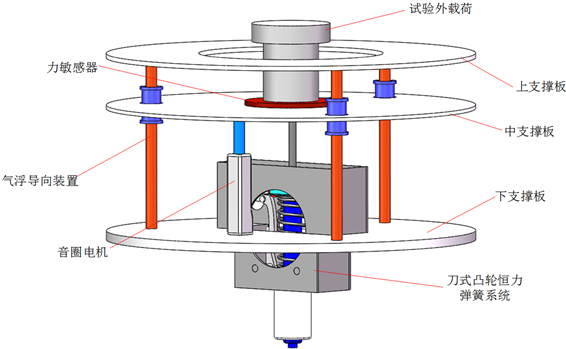Figure 1. Schematic diagram of electromechanical composite constant force spring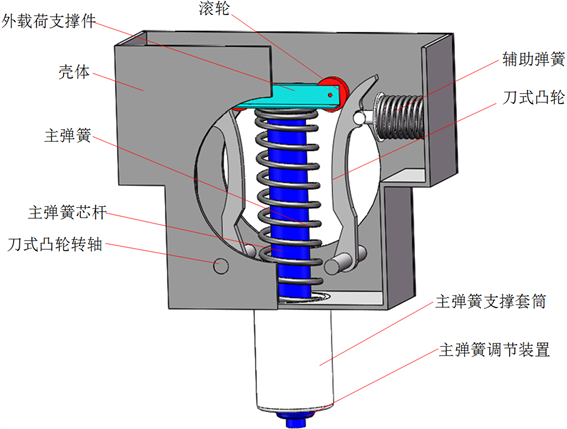Figure 2. Schematic diagram of mechanical passive constant force system

3. 机械式被动恒力系统建模与分析

3.1. 机械式被动恒力系统建模

3.2. 试验设计Table 1. Blade cam constant force spring test design table

3.3. 试验结果分析

${\text{e}}^{\left(i\right)}={F}_{out}{}^{\left(i\right)}-G$ (1)

Fout的平均值 $\mu =0.2567$，标准差 $\sigma =1.1337$，且输出力误差概率密度近似呈正态分布，根据3 $\sigma$ 准则，则输出力误差范围为：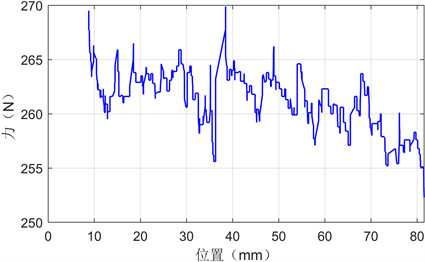Figure 3. Test result curve

${e}_{\text{t}}=\left[\mu -3\sigma ,\mu +3\sigma \right]=\left[\text{-3}\text{.1444},\text{3}\text{.6578}\right]$ (2)

3.4. 系统辨识结果与误差分析

$MSE=\underset{\text{i}=1}{\overset{500}{\sum }}{\left({\stackrel{^}{\theta }}_{\text{i}}-{\theta }_{\text{i}}\right)}^{2}/500$ (3)Table 2. Comparison table of identification results of three neural network algorithms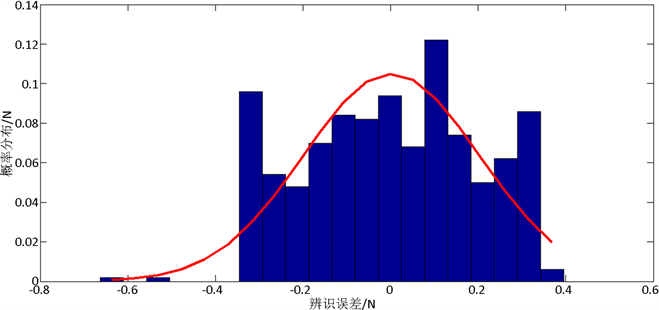Figure 4. Fuzzy neural network system identification error probability density map

4. 机电复合式恒力弹簧仿真与分析

4.1. 控制系统设计

PID由于其参数较少，较易整定且便于学习与操作，在工业控制领域应用非常广泛，但是PID算法在误差变化快速、非线性等系统当中难以适应，且PID控制也无法满足高精度控制的要求。所以，直线电机的控制系统可基于模型参考神经网络控制系统思想进行设计。模型参考神经网络控制方法(Neural Network Model Reference Adaptive Control, NNMRAC)由国外学者提出，是一种将神经网络控制与模型参考自适应控制相结合的一种新的控制方法 。

4.2. 机电复合式恒力弹簧仿真设计

4.2.1 . 参考模型设计

${F}_{e}\left(s\right)=\frac{U\left(s\right)-\frac{{K}_{e}{v}_{e}}{s}}{Ls+R}{K}_{e}$ (4)

${F}_{e}\left(n\right)={K}_{e}\frac{\Delta t\cdot {u}_{e}\left(n\right)-\Delta t\cdot {K}_{e}\cdot {v}_{e}\left(n-1\right)+L\cdot i\left(n-1\right)}{\Delta t\cdot R+L}$ (5)

$\Delta t$ ——时间步长(s)；

u——电枢端电压(V)；

n——迭代次数。

$\frac{{F}_{e}\left(n\right)\cdot \left(\Delta t\cdot R+L\right)/{K}_{e}+\Delta t\cdot {K}_{e}\cdot {v}_{e}\left(n-1\right)-L\cdot i\left(n-1\right)}{\Delta t}$ (6)

${u}_{e}=\left\{\begin{array}{ll}+72\hfill & {u}_{e}>72\hfill \\ {u}_{e}\hfill & -72\le {u}_{e}\le +72\hfill \\ -72\hfill & {u}_{e}<-72\hfill \end{array}$ (7)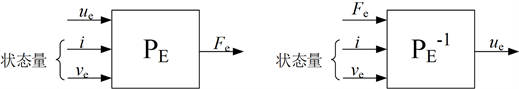Figure 5. Reference model and its inverse model

4.2.2 . 神经网络控制器和辨识器设计

4.2.3 . 控制对象模型设计

$\begin{array}{c}{R}^{\prime }=K{}_{1}R\\ {L}^{\prime }={K}_{2}L\\ {{K}^{\prime }}_{e}={K}_{3}{K}_{e}\end{array}$ (8)

${F}_{m}\left(n\right)={{K}^{\prime }}_{e}\frac{\Delta t\cdot {{u}^{\prime }}_{m}\left(n\right)-\Delta t\cdot {{K}^{\prime }}_{e}\cdot {v}_{e}\left(n-1\right)+{L}^{\prime }\cdot i\left(n-1\right)}{\Delta t\cdot {R}^{\prime }+{L}^{\prime }}$ (9)

${{u}^{\prime }}_{m}$ ——控制对象模型输入量(V)。

4.2.4 . 系统总体模型设计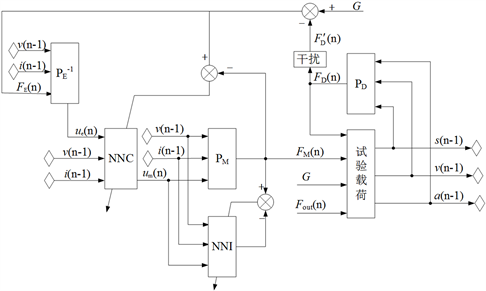Figure 6. Electromechanical hybrid constant force system overall simulation model

4.3. 仿真结果分析

4.3.1 . 被控对象模型与参考模型差异的影响Table 3. Controlled object model hardware parameter impact test group table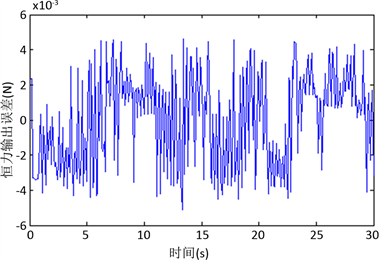Figure 7. Constant force output after adding armature terminal voltage noise

4.3.2 . 试验载荷运动状态的影响Table 4. Test load movement state influence test group table

5. 结束语

1) 对机械式被动恒力系统以及电磁式主动力补偿系统进行了设计，并且对其误差的主要来源进行了分析。

2) 对所设计的机械式被动恒力系统建立等价数学模型，基于试验数据利用神经网络对机械式被动恒力系统进行了辨识，并与对照组数据进行比较，验证了等价模型的正确性，且在该模型的基础上施加主动控制并进行仿真和分析。

3) 选择模型参考神经网络控制系统，通过对控制系统进行建模和仿真，分别评估了被控对象模型与参考模型之间的差异以及试验载荷运动状态对控制的影响。试验结果表明，模型差异对恒力输出影响很小，试验载荷运动的动态性越高，系统恒力输出的误差率也越高，但总体量级仍然较小，满足0.5%的预定误差指标，且比1.42%的机械式恒力系统误差要小的多，系统设计及控制效果得到了验证。

  Matunaga, S., Yoshihara, K., Takahashi, T., et al. (2000) Ground Experiment System for Dual-Manipulator-Based Capture of Damaged Satellites. IEEE/RSJ International Conference on Intelligent Robots and Systems, Takamatsu, 31 Octo-ber-5 November 2000, 1847-1852.  李志军. 五自由度模拟器关键机械结构设计及控制系统分析[D]: [硕士学位论文]. 哈尔滨: 哈尔滨工业大学, 2015: 8-14.  白晓辉, 白鸿柏, 郝慧荣, 刘远方. 正负刚度并联机构动力学分析及隔振仿真[J]. 机械工程师, 2009(11): 87-89.  张勇. 机电复合式恒力系统设计与分析[D]: [硕士学位论文]. 哈尔滨: 哈尔滨工业大学, 2018.  Moody, J. and Darken, C.J. (2014) Fast Learning in Networks of Locally-tuned Processing Units. Neural Computation, 1, 281-294. https://doi.org/10.1162/neco.1989.1.2.281  Skaf, Z., Wang, H. and Guo, L. (2011) Fault Tolerant Control Based on Stochastic Distribution via RBF Neural Networks. Journal of Systems Engineering and Electronics, 22, 63-69. https://doi.org/10.3969/j.issn.1004-4132.2011.01.007  乔俊飞. 前馈神经网络分析与设计[M]. 北京: 科学出版社, 2013: 23-31.  Jyh-Shing, R.J. (1993) Adaptive-Network-Based Fuzzy Inference System. IEEE Transactions on Systems, Man, and Cybernetics, 23, 665-685. https://doi.org/10.1109/21.256541